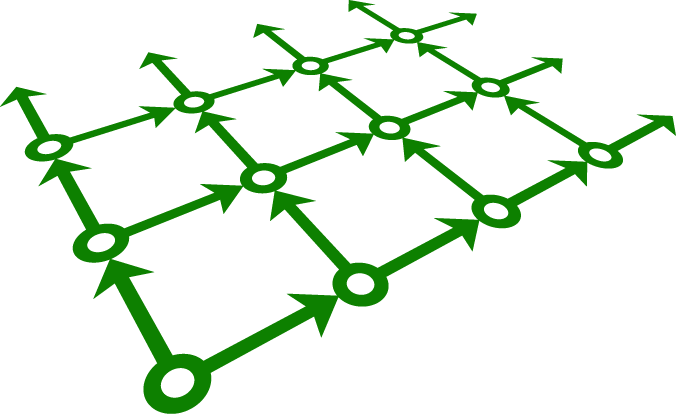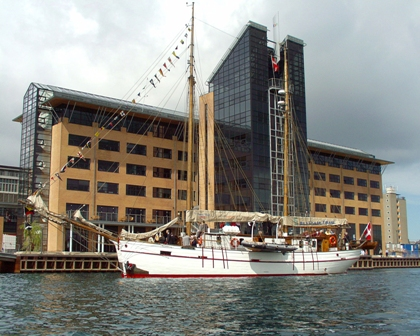Topics

We invite submission on all aspects of modal logic, including:
• history of modal logic
• philosophy of modal logic
• applications of modal logic
• computational aspects of modal logic
• complexity and decidability of modal and temporal logics
• modal and temporal logic programming
• model checking
• model generation
• theorem proving for modal logics
• theoretical aspects of modal logic
• algebraic and categorical perspectives on modal logic
• coalgebraic modal logic
• completeness and canonicity
• correspondence and duality theory
• many-dimensional modal logics
• modal fixed point logics
• model theory of modal logic
• proof theory of modal logic
• specific instances and variations of modal logic
• description logics
• dynamic logics and other process logics
• epistemic and deontic logics
• modal logics for agent-based systems
• modal logic and game theory
• modal logic and grammar formalisms
• provability and interpretability logics
• spatial and temporal logics
• hybrid logic
• intuitionistic logic
• substructural logics
• computationally light fragments of all such logics

Papers on related subjects will also be considered.

Special sessions

AiML 2012 will host three special sessions. Papers for each of them should be submitted to the EasyChair site in the same way as other papers.

Special session on hybrid logic

Hybrid logic is an extension of modal logic which allows us to refer explicitly to states of the model in the syntax of formulas. There will be a special session of AiML 2012 devoted to papers on hybrid logic.

The scope of the special session is standard hybrid-logical machinery like nominals, satisfaction operators, and the downarrow binder, but also other extensions of modal logic can be considered.

Special session in honour of Larisa Maksimova

There will be a special session of AiML devoted to papers and talks in honour of Larisa Maksimova, in recognition of her many outstanding contributions to modal logic.